Скачать презентацию Chapter 3 ANNUITIES Basic Results Perpetuities

• Количество слайдов: 21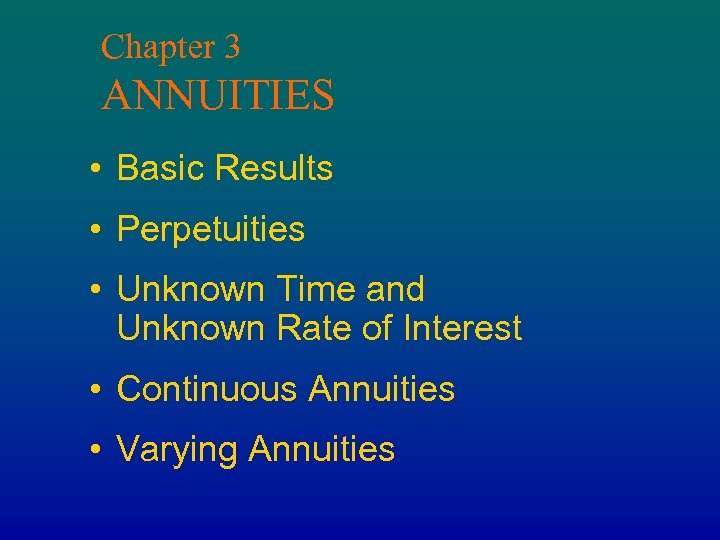Chapter 3 ANNUITIES • Basic Results • Perpetuities • Unknown Time and Unknown Rate of Interest • Continuous Annuities • Varying Annuities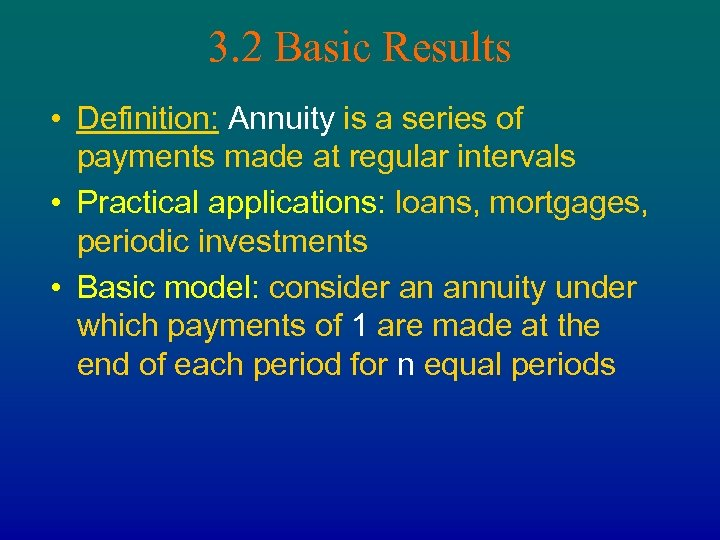3. 2 Basic Results • Definition: Annuity is a series of payments made at regular intervals • Practical applications: loans, mortgages, periodic investments • Basic model: consider an annuity under which payments of 1 are made at the end of each period for n equal periods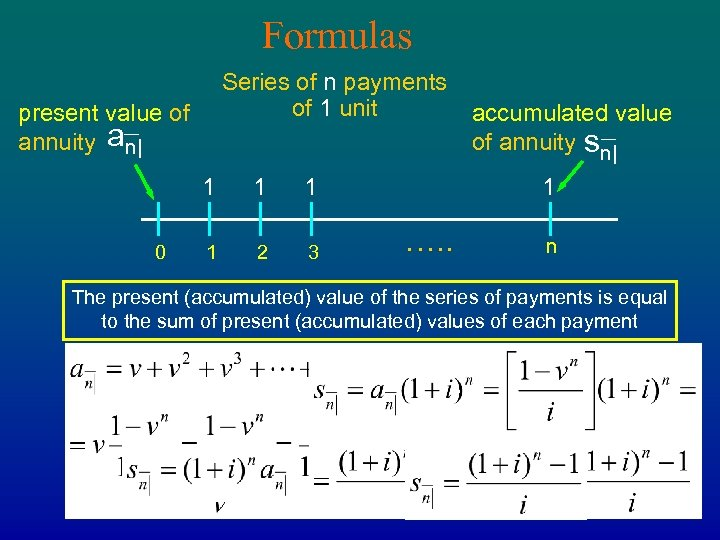Formulas Series of n payments of 1 unit present value of annuity an| 1 0 1 1 1 2 3 accumulated value of annuity sn| 1 …. . n The present (accumulated) value of the series of payments is equal to the sum of present (accumulated) values of each payment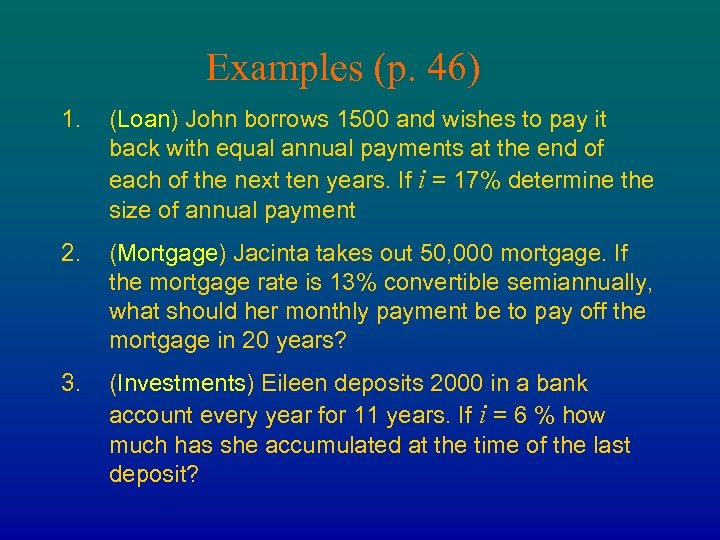Examples (p. 46) 1. (Loan) John borrows 1500 and wishes to pay it back with equal annual payments at the end of each of the next ten years. If i = 17% determine the size of annual payment 2. (Mortgage) Jacinta takes out 50, 000 mortgage. If the mortgage rate is 13% convertible semiannually, what should her monthly payment be to pay off the mortgage in 20 years? 3. (Investments) Eileen deposits 2000 in a bank account every year for 11 years. If i = 6 % how much has she accumulated at the time of the last deposit?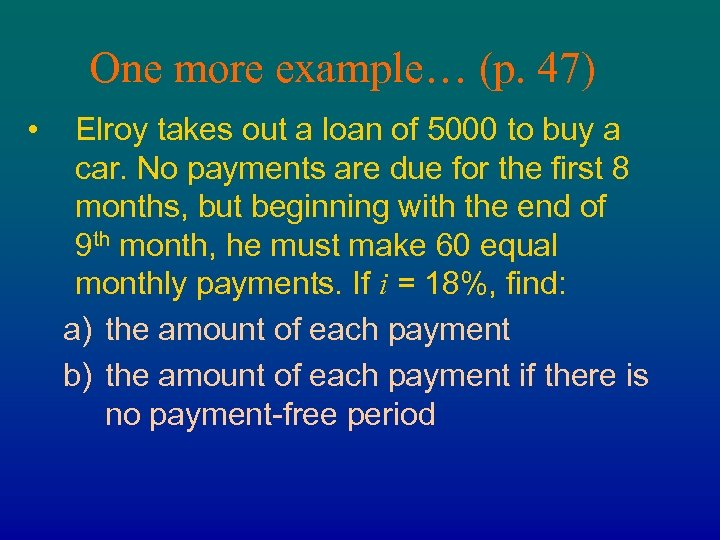One more example… (p. 47) • Elroy takes out a loan of 5000 to buy a car. No payments are due for the first 8 months, but beginning with the end of 9 th month, he must make 60 equal monthly payments. If i = 18%, find: a) the amount of each payment b) the amount of each payment if there is no payment-free periodAnnuity-immediate and Annuity-due an| Annuity-immediate (payments made at the end) 1 1 1 0 än| 1 2 3 …. . sn| 1 n . . sn| Annuity-due (payments made at the beginning) 1 1 2 3 1 …. . n n+1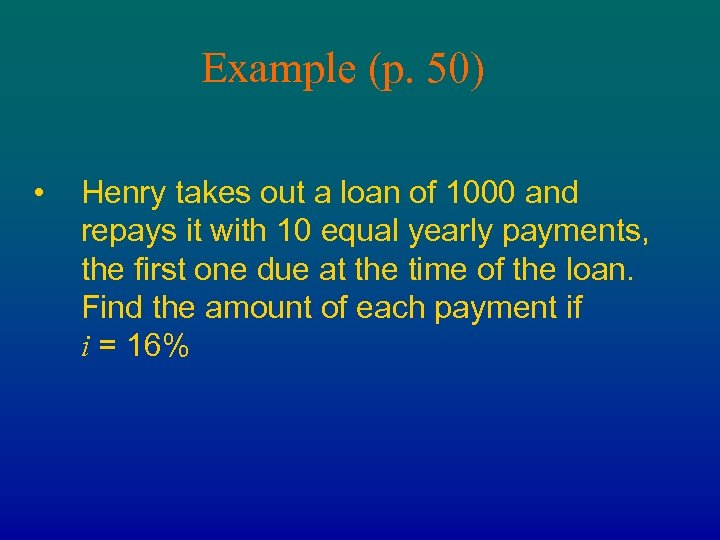Example (p. 50) • Henry takes out a loan of 1000 and repays it with 10 equal yearly payments, the first one due at the time of the loan. Find the amount of each payment if i = 16%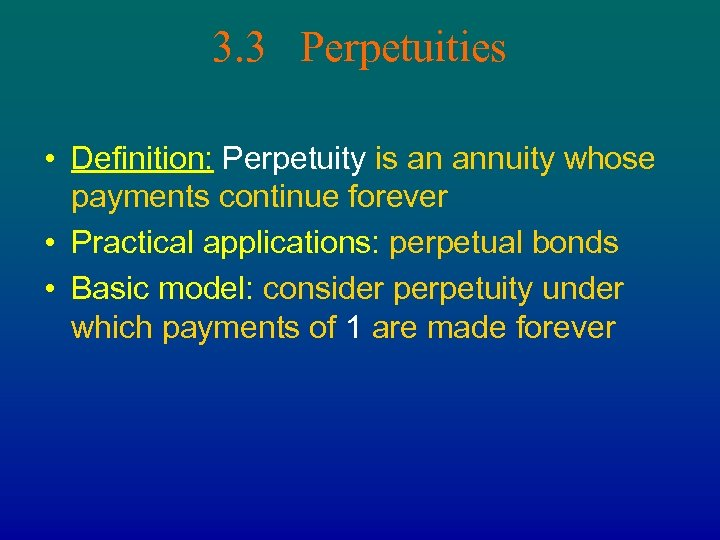3. 3 Perpetuities • Definition: Perpetuity is an annuity whose payments continue forever • Practical applications: perpetual bonds • Basic model: consider perpetuity under which payments of 1 are made forever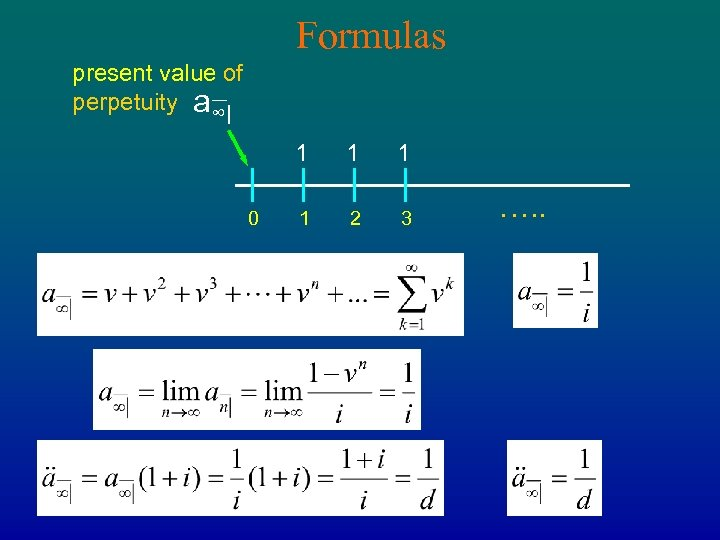Formulas present value of perpetuity a∞| 1 0 1 1 1 2 3 …. .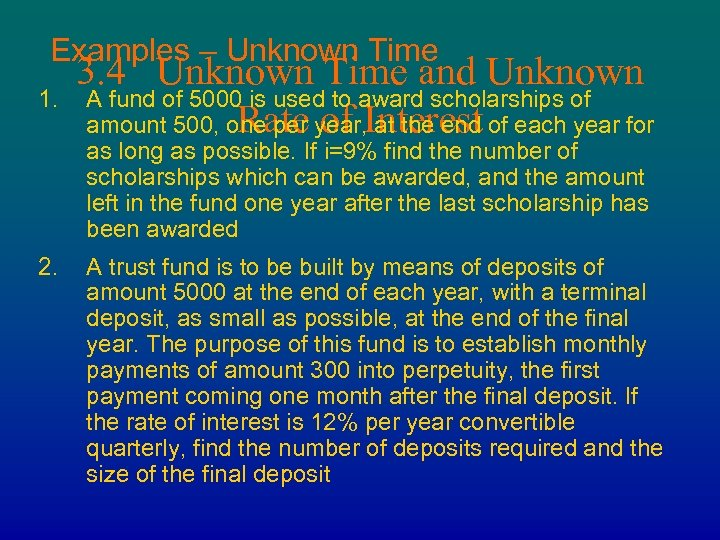Examples – Unknown Time 1. 3. 4 Unknown Time and Unknown A fund of 5000 is used to award scholarships of Rate of at the end amount 500, one per year, Interest of each year for as long as possible. If i=9% find the number of scholarships which can be awarded, and the amount left in the fund one year after the last scholarship has been awarded 2. A trust fund is to be built by means of deposits of amount 5000 at the end of each year, with a terminal deposit, as small as possible, at the end of the final year. The purpose of this fund is to establish monthly payments of amount 300 into perpetuity, the first payment coming one month after the final deposit. If the rate of interest is 12% per year convertible quarterly, find the number of deposits required and the size of the final deposit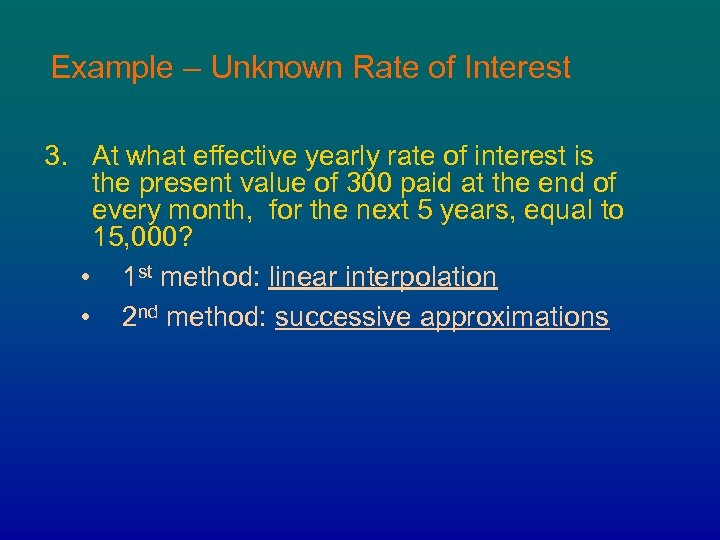Example – Unknown Rate of Interest 3. At what effective yearly rate of interest is the present value of 300 paid at the end of every month, for the next 5 years, equal to 15, 000? • 1 st method: linear interpolation • 2 nd method: successive approximations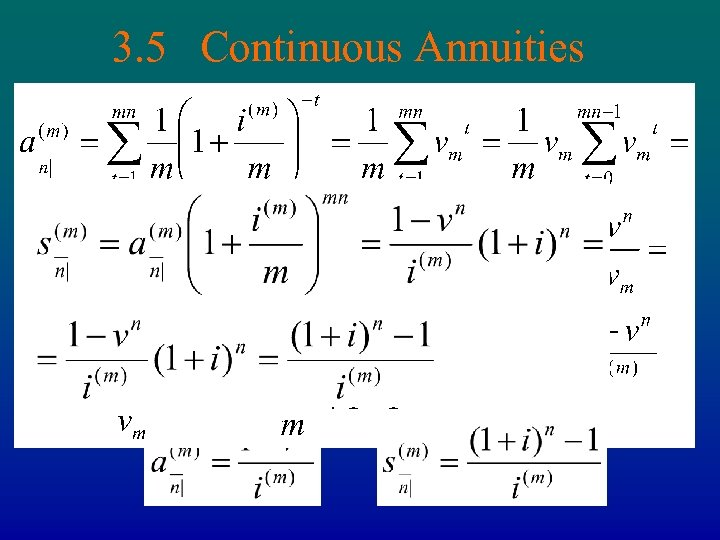3. 5 Continuous Annuities Let effective period be 1/m part of the year and i(m)/m be the effective rate of interest: (1+ i(m)/m)m = 1 + i Series of n∙m payments of 1/m 1/m 1/m 0 1/m 2/m 3/m …. . Formula: n = nm(1/m)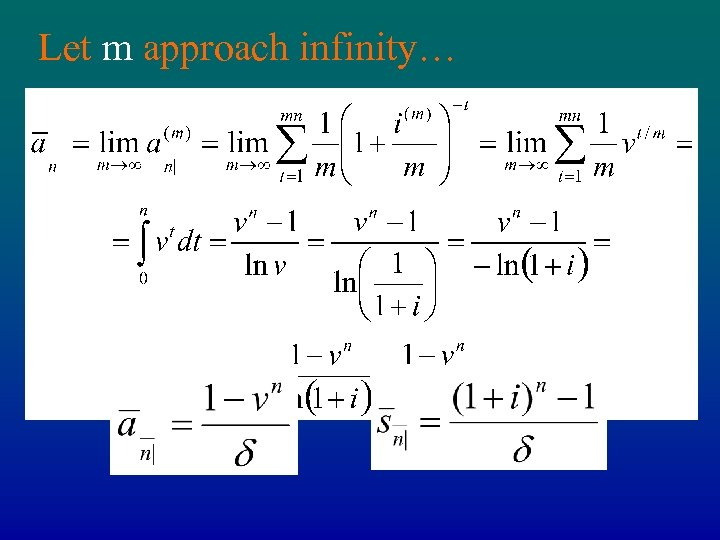Let m approach infinity… • Present value of continuous annuity • Accumulated value of continuous annuity Formulas: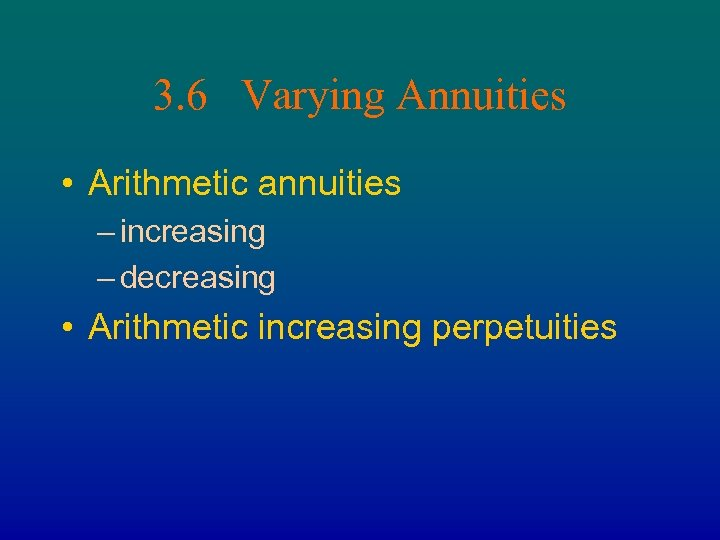3. 6 Varying Annuities • Arithmetic annuities – increasing – decreasing • Arithmetic increasing perpetuities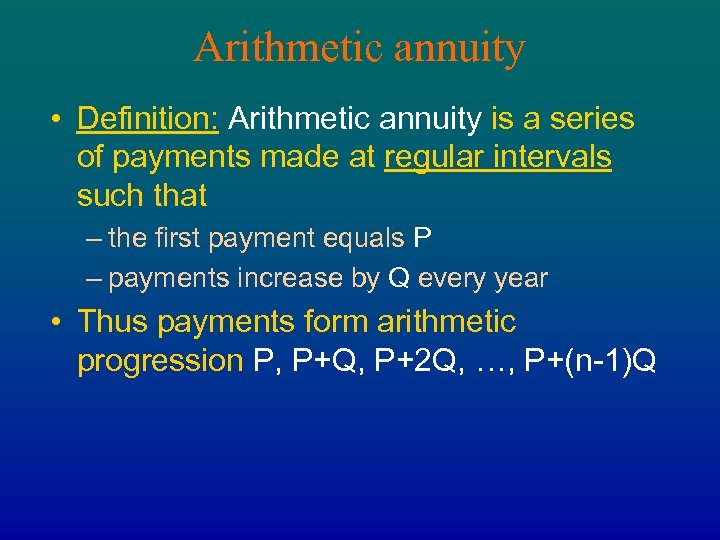Arithmetic annuity • Definition: Arithmetic annuity is a series of payments made at regular intervals such that – the first payment equals P – payments increase by Q every year • Thus payments form arithmetic progression P, P+Q, P+2 Q, …, P+(n-1)Q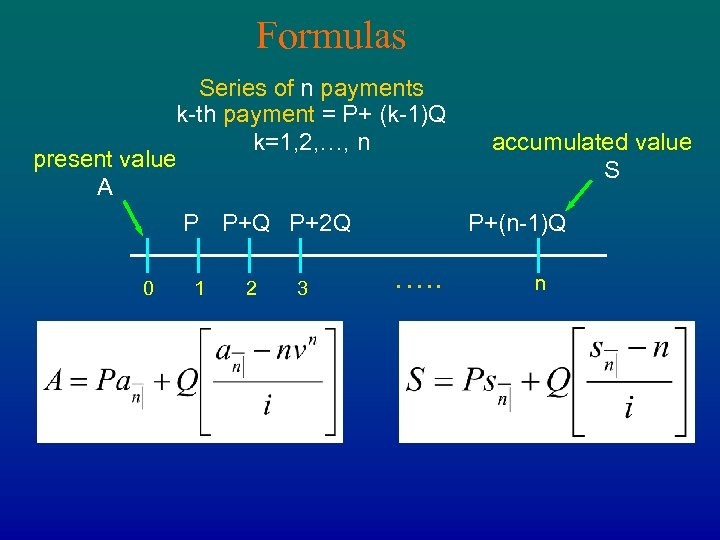Formulas Series of n payments k-th payment = P+ (k-1)Q accumulated value k=1, 2, …, n present value S A P P+Q P+2 Q P+(n-1)Q 0 1 2 3 …. . n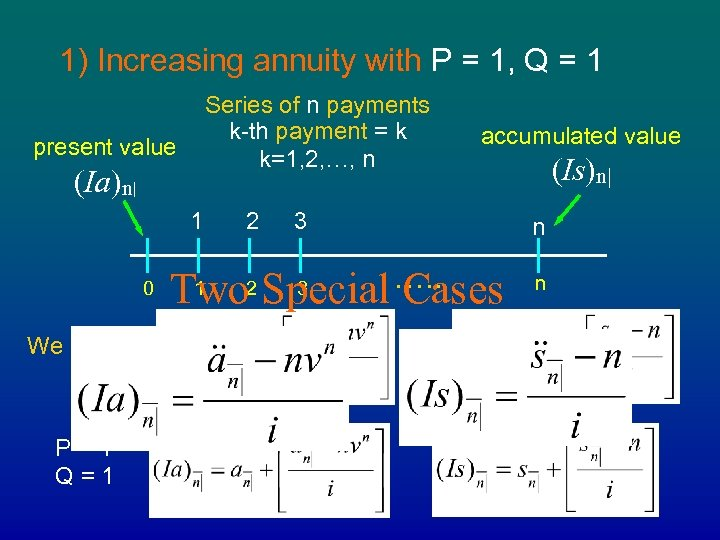1) Increasing annuity with P = 1, Q = 1 Series of n payments k-th payment = k k=1, 2, …, n present value (Ia)n| 1 0 We have: P=1 Q=1 2 Two 1 2 accumulated value 3 …. . Special Cases 3 (Is)n| n n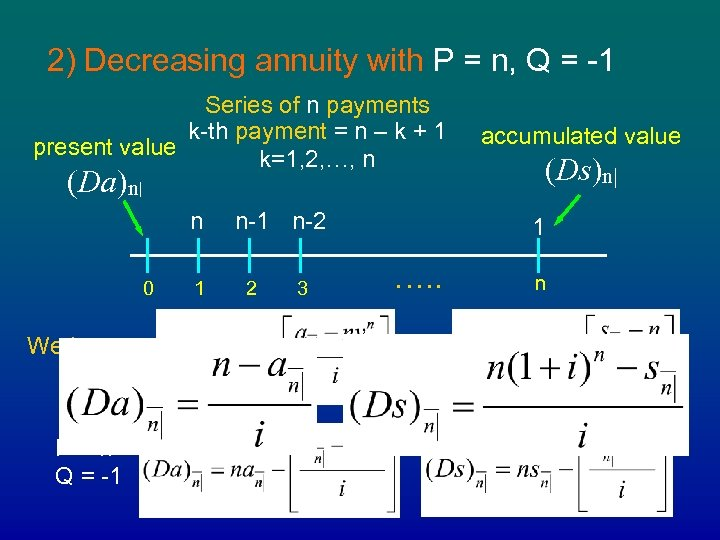2) Decreasing annuity with P = n, Q = -1 Series of n payments k-th payment = n – k + 1 present value k=1, 2, …, n (Da)n| n 0 We have: P=n Q = -1 1 n-2 2 3 accumulated value (Ds)n| 1 …. . n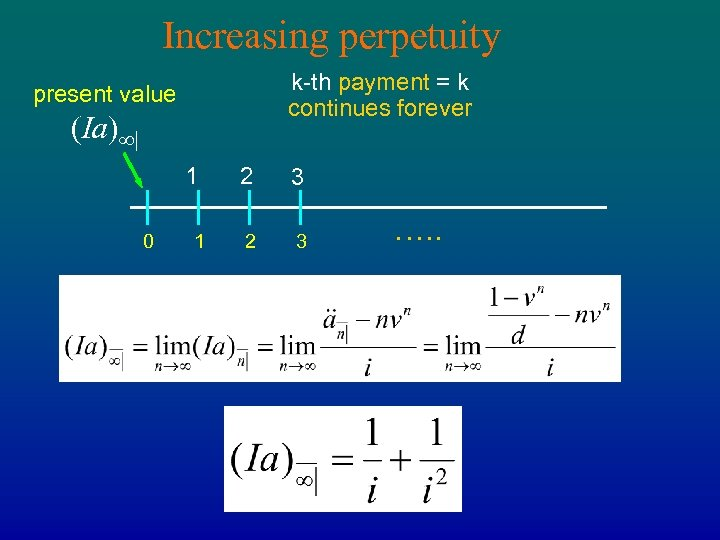Increasing perpetuity k-th payment = k continues forever present value (Ia)∞| 1 0 1 2 3 …. .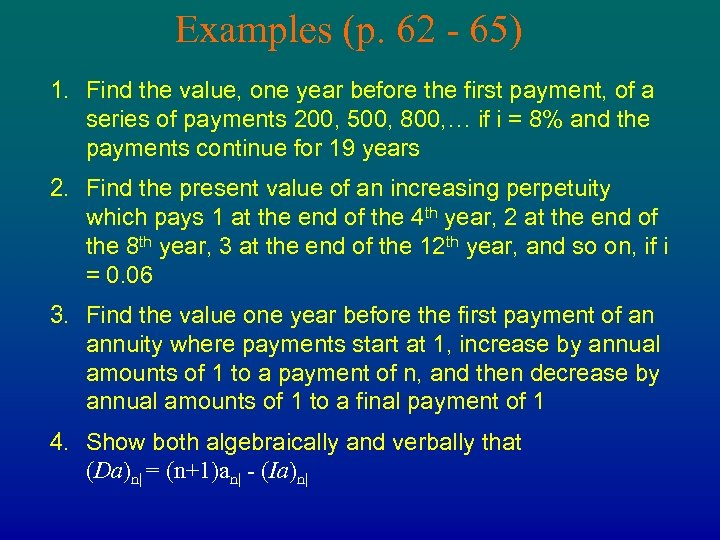Examples (p. 62 - 65) 1. Find the value, one year before the first payment, of a series of payments 200, 500, 800, … if i = 8% and the payments continue for 19 years 2. Find the present value of an increasing perpetuity which pays 1 at the end of the 4 th year, 2 at the end of the 8 th year, 3 at the end of the 12 th year, and so on, if i = 0. 06 3. Find the value one year before the first payment of an annuity where payments start at 1, increase by annual amounts of 1 to a payment of n, and then decrease by annual amounts of 1 to a final payment of 1 4. Show both algebraically and verbally that (Da)n| = (n+1)an| - (Ia)n|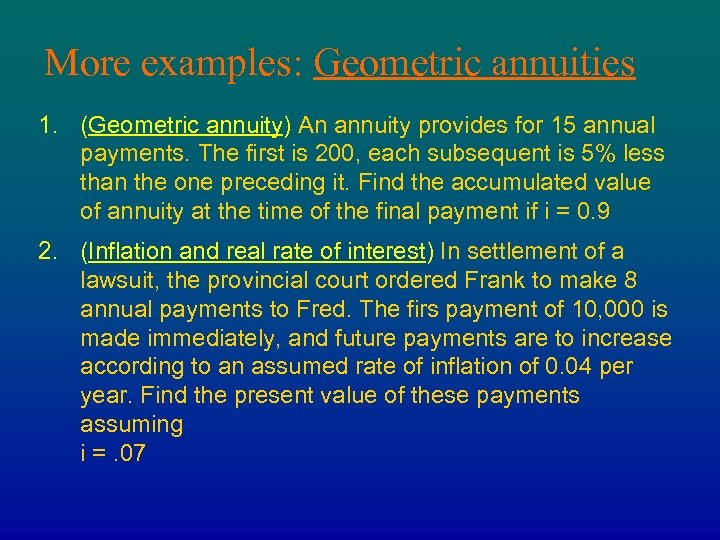More examples: Geometric annuities 1. (Geometric annuity) An annuity provides for 15 annual payments. The first is 200, each subsequent is 5% less than the one preceding it. Find the accumulated value of annuity at the time of the final payment if i = 0. 9 2. (Inflation and real rate of interest) In settlement of a lawsuit, the provincial court ordered Frank to make 8 annual payments to Fred. The firs payment of 10, 000 is made immediately, and future payments are to increase according to an assumed rate of inflation of 0. 04 per year. Find the present value of these payments assuming i =. 07